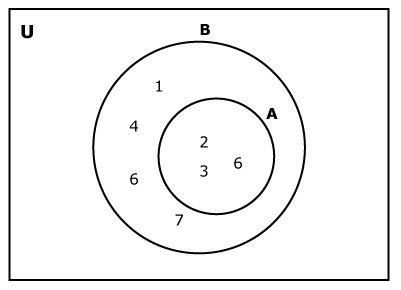# Python Set issubset()

The issubset() method returns True if all elements of a set are present in another set (passed as an argument). If not, it returns False.

Set A is said to be the subset of set B if all elements of A are in B .Here, set A is a subset of B.

The syntax of issubset() is:

`A.issubset(B)`

The above code checks if A is a subset of B.

## Return Value from issubset()

The issubset() returns

• True if A is a subset of B
• False if A is not a subset of B

## Example: How issubset() works?

```A = {1, 2, 3}
B = {1, 2, 3, 4, 5}
C = {1, 2, 4, 5}

# Returns True
print(A.issubset(B))

# Returns False
# B is not subset of A
print(B.issubset(A))

# Returns False
print(A.issubset(C))

# Returns True
print(C.issubset(B))
```

When you run the program, the output will be:

```True
False
False
True```

If you need to check if a set is a superset of another set, you can use issuperset() in Python.